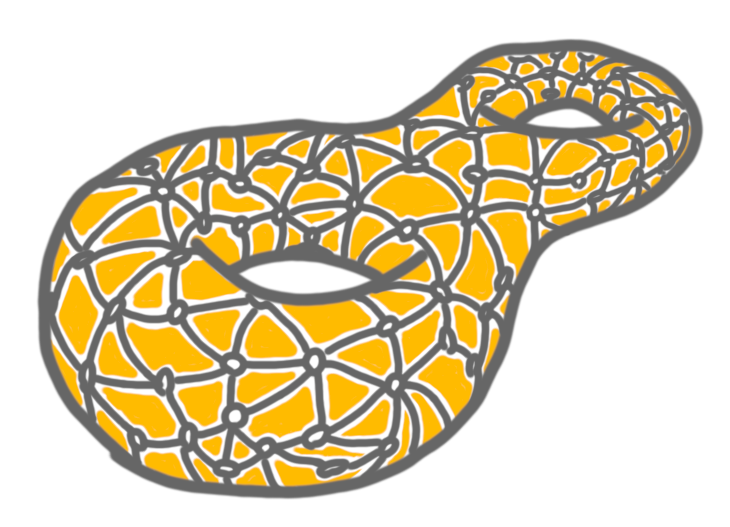# Local Certification of Graphs with Bounded Genus

Discrete Applied Mathematics.

doi:10.1016/j.dam.2022.10.004Naor, Parter, and Yogev [SODA 2020]~recently designed a compiler for automatically translating standard centralized interactive protocols to distributed interactive protocols, as introduced by Kol, Oshman, and Saxena [PODC 2018]. In particular, by using this compiler, every linear-time algorithm for deciding the membership to some fixed graph class can be translated into a $MAM(O(\log n))$ protocol for this class, that is, a distributed interactive protocol with $O(\log n)$-bit proof size in $n$-node graphs, and three interactions between the (centralized) computationally-unbounded but non-trustable prover Merlin, and the (decentralized) randomized computationally-limited verifier Arthur. As a corollary, there is a $MAM(O(\log n))$ protocol for recognizing the class of planar graphs, as well as for recognizing the class of graphs with bounded genus.
We show that there exists a distributed interactive protocol for recognizing the class of graphs with bounded genus performing just a single interaction, from the prover to the verifier, yet preserving proof size of $O(\log n)$ bits. This result also holds for the class of graphs with bounded non-orientable genus, that is, graphs that can be embedded on a non-orientable surface of bounded genus. The interactive protocols described in this paper are actually proof-labeling schemes, i.e., a subclass of interactive protocols, previously introduced by Korman, Kutten, and Peleg [PODC 2005]. In particular, these schemes do \emph{not} require any randomization from the verifier, and the proofs may often be computed a priori, at low cost, by the nodes themselves. Our results thus extend the recent proof-labeling scheme for planar graphs by Feuilloley et al. [PODC 2020], to graphs of bounded genus, and to graphs of bounded non-orientable genus.